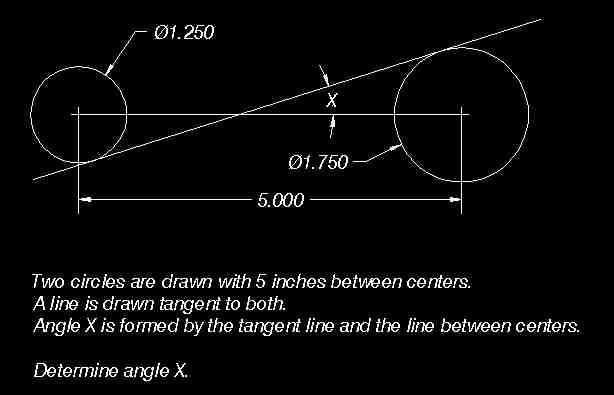# Trig Problem - Way Overthinking This

## Homework Statement## Homework Equations

Not sure, thought of everything from finding the length of the transverse common tangent of the circles, to staring at the Inscribed Angle Theorem for a while...

## The Attempt at a Solution

I believe I'm overthinking this problem, at first I tried to find a way of figuring out the slope of the transverse common tangent, but the figures aren't on a coordinate plane, so I'm not sure how I would go about it, or even if that's needed to solve the problem.

Then I gave some thought to finding the radii of the two circles based off the length of the inner tangent, which is given as 5 inches, though I'm not sure how that would help either.

I suppose I just need a hint in the right direction, this seems so simple, but for some reason the solution just isn't clicking.

## The Attempt at a Solution

vela
Staff Emeritus
Homework Helper
Try drawing lines connecting the center of each circle to the point where the line is tangent to the circle. Contemplate the two triangles you just formed.

Okay, so I now have two scalene triangles that I can prove are similar using the Angle Side Angle postulate, and their shared side sums to the measure of the inner tangent, 5 inches, though I can't see how I can derive the actual measures from this.

I've been taking the values of θ given for the measure of the central angle, but using these two bits of information I still can't seem to figure out a way to solve for x.

vela
Staff Emeritus
Homework Helper
What are the lengths of the line segments you drew? Also, what's the angle between those lines and the tangent line?

Alright, I feel a tad stupid. I was taking the symbol in front of 1.25 and 1.75 as a theta, not the symbol for diameter. Makes things a bit easier, for the life of me I couldn't figure out how to find the radii.I've now got something similar to this:

http://img517.imageshack.us/img517/4583/triganles.jpg [Broken]

Am I on the right track?

Last edited by a moderator:
vela
Staff Emeritus
Homework Helper
Yes. Now you can use the fact they are similar triangles to calculate the hypotenuse of either triangle.

Alright, I used the ratio between the two triangles to figure out the length of each hypotenuse, plugged everything into Pythagoras' theorem to find the measure of the two opposite angles, then everything went into the Law of Sines to find the measure of x, which I believe to be roughly 25 degrees.

vela
Staff Emeritus
Homework Helper
I got a different answer. What did you get for the hypotenuse of the triangles?

For the hypotenuse of the smaller triangle, ~1.43", for the larger triangle, ~3.57".

vela
Staff Emeritus
Homework Helper
I got 25/12 and 35/12. What equations did you get when you solved for them?

Just rechecked my work, I had written down 25/12 and 35/12 on paper, but when I solved for the angles I managed to plug in two fractions from a different problem into my calc.

Edit: I also used the value of sin(θ), instead of sin^-1(sin(θ)), for the degrees.

vela
Staff Emeritus
Mad props dude, without your help, I'd still be slamming my head against my desk trying to figure this out.